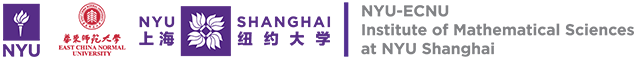# Centers & InstitutesThe NYU-ECNU Institute of Mathematical Sciences at NYU Shanghai is dedicated to the development of a unique research and training environment to advance modern mathematics and its applications. The Institute works in close partnership with New York University’s Courant Institute of Mathematical Sciences and East China Normal University’s Department of Mathematics.

Research work at the Institute is patterned after the style of mathematics that has become the trademark of the Courant Institute since its founding in 1935: strong, dedicated and talented mathematicians working on problems of important scientiﬁc, technological, social, or economic consequences and developing the mathematical and computational methods to solve these problems. The research interests at the Institute include theories and applications of probability, nonlinear partial diﬀerential equations, ﬂuid dynamics, biology, materials science, computational neuroscience, and mathematical ﬁnance.

The Department of Mathematics at ECNU was founded in 1951. In 1996 the department was listed as one of the national bases in China for basic research in science and talent training in mathematics. The Department of Mathematics has strengths in pure mathematics, applied mathematics, computational mathematics, and math education. The Department is an inﬂuential math research and education base both in and out of China.

The Institute aspires to attract scientiﬁc and mathematic talents to Shanghai.